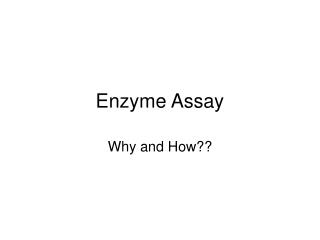DownloadDownload PresentationEnzyme Assay

# Enzyme Assay

Download Presentation## Enzyme Assay

- - - - - - - - - - - - - - - - - - - - - - - - - - - E N D - - - - - - - - - - - - - - - - - - - - - - - - - - -
##### Presentation Transcript

1. Enzyme Assay Why and How??

2. Introduction • In most cases, actual molar enzyme concentration is not known. We measure amount of enzyme by its activity in terms of enzyme unit of rate or a IU. • IU is also a way to monitor the enzyme purification. • In our study the enzyme activity we measure is more related to amount of enzyme rather than its physiological situation.

3. IU • One IU is defined as the amount of enzyme that produces a μmole product per minute under defined conditions. • The concentration of enzyme is expressed as IU/mL • The specific activity (SA) is the number of IU per mg protein. • As the enzyme is purified the SA increases

4. Classical Chemical Kinetics • Classical kinetic theory answers the question “what determines the rate of formation or destruction of any given compound?” • k 1 • A + B → C +D • K-1 • The rate of formation of one compound (lets say C) at any time (dC/dt) is proportional to the concentration of the reactants (A, B) and the rate constant (k1). • Formation of

5. Enzyme Kinetics • Recent methods invovle enzyme-substrate complex formation Enzyme + Substrate → Enzyme substrate Complex → Enzyme + Product Michaelis-Menten Equation Vo = Vmax[S] / Km – [S] Vo = initial reaction ; [S] = substrate concentration; km affinity between enzyme and substrate Initial stages of reaction is dependent on substrate concentration and is the fastest reaction at any given enzyme concentrations

6. Initial velocity • dp/dt = [ES]k2 … no backward reaction • Total enzyme [Enztotal] = [Enzfree] + [ES] • The first assumption is true only for initial stages of reaction. • Vo - Fastest and dependent on substrate concentration. • ES ~ E total • Vo =[Enzyme]k2 = Vmax

7. Initial reactions • If we have excess substrate , initial velocity is always a function of the total enzyme. • Vo will be different at different enzyme concentrations. • If we plot Vo versus time, one should get straight line.

8. Enzyme activity • Protein assay: to get total protein (crude to pure there will be a decrease in enzyme –non-enzyme protein ratio) • IU by using initial velocities • G3P + NAD + iP → 1,3 BPG + NADH + H+ • The kinetics follows rapid equilibrium random bi bi mechanism. The reaction is first order in the total amount of enzyme.

9. Measuring the product • Formation of NADH. • Method 1: Generate G3P by mixing FDP and aldolase which then under goes the rxn • Method2: Use commercial G3P (expensive and unstable)